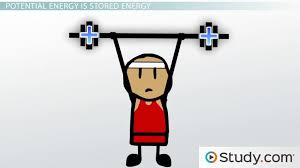Work and Power
3 years ago
lisatusa32
Save
Edit
Host a game
Live GameLive
Homework
Solo Practice
Practice10 QuestionsShow answers
• Question 1
30 seconds
Q. Work divided by time equals...
energy
power
• Question 2
30 seconds
Q. The unit of work is the ...
joule
force
• Question 3
30 seconds
Q. The unit of power is the...
work
watt
• Question 4
30 seconds
Q. Force multiplied by distance equals...
work
power
• Question 5
30 seconds
Q. Work is done on an object when the object moves in the SAME direction in which the force is applied.
True
False
• Question 6
30 seconds
Q. If you pull at an angle instead of in the direction in which the object moves, MORE of your force does work.
True
False
• Question 7
30 seconds
Q. The FARTHER you move an object, the more work you do.
True
False
• Question 8
30 seconds
Q. The more POWER you use to move an object, the more work you do.
True
False
• Question 9
30 seconds
Q. If you lift a box from the floor to a height of 1m and then carry the for for 10m, you do work only when you CARRY the box.